Open in App
Not now

# Pointer Expressions in C with Examples

• Difficulty Level : Medium
• Last Updated : 21 Feb, 2023

Prerequisite: Pointers in C Pointers are used to point to address the location of a variable. A pointer is declared by preceding the name of the pointer by an asterisk(*). Syntax:

`datatype *pointer_name;`

When we need to initialize a pointer with variable’s location, we use ampersand sign(&) before the variable name. Example:

## C

 `// Declaration of integer variable` `int` `var=10;`   `// Initialization of pointer variable` `int` `*pointer=&var;`

The ampersand (&) is used to get the address of a variable. We can directly find the location of any identifier by just preceding it with an ampersand(&) sign. Example:

## C

 `// This code prints the address of x` `#include `   `int` `main()` `{` `    ``int` `x = 10;`   `    ``// Prints address of x` `    ``printf``(` `        ``"Address of variable x = %p", &x);`   `    ``return` `0;` `}`

Output:

`Address of variable x = 0x7fff3b690fd4`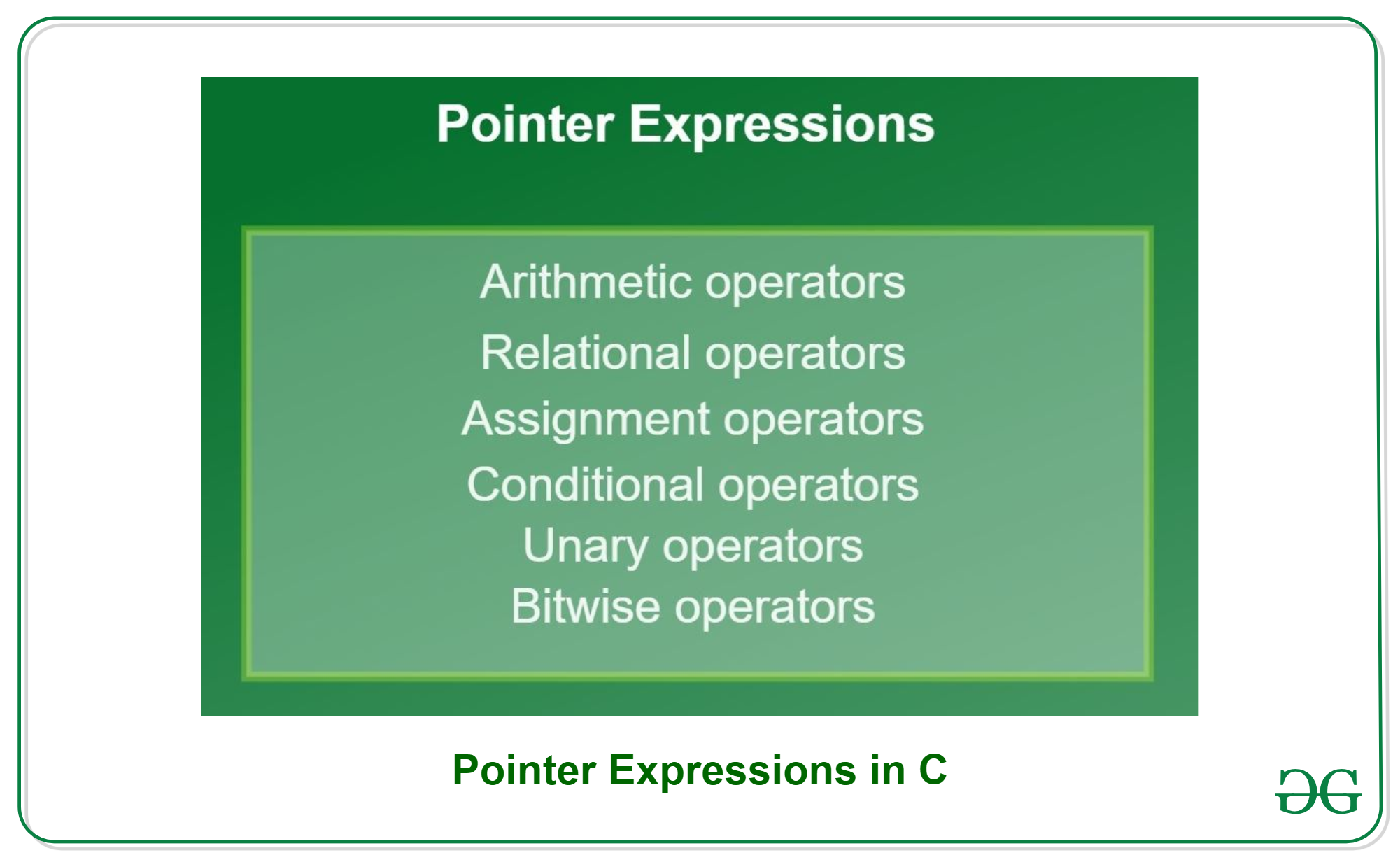C supports a rich set of built-in operations like arithmetic, relational, assignment, conditional, etc. which can be performed on identifiers. Just like any other variable, these operations can be also performed on pointer variables.

Arithmetic Operators

We can perform arithmetic operations to pointer variables using arithmetic operators. We can add an integer or subtract an integer using a pointer pointing to that integer variable. The given table shows the arithmetic operators that can be performed on pointer variables: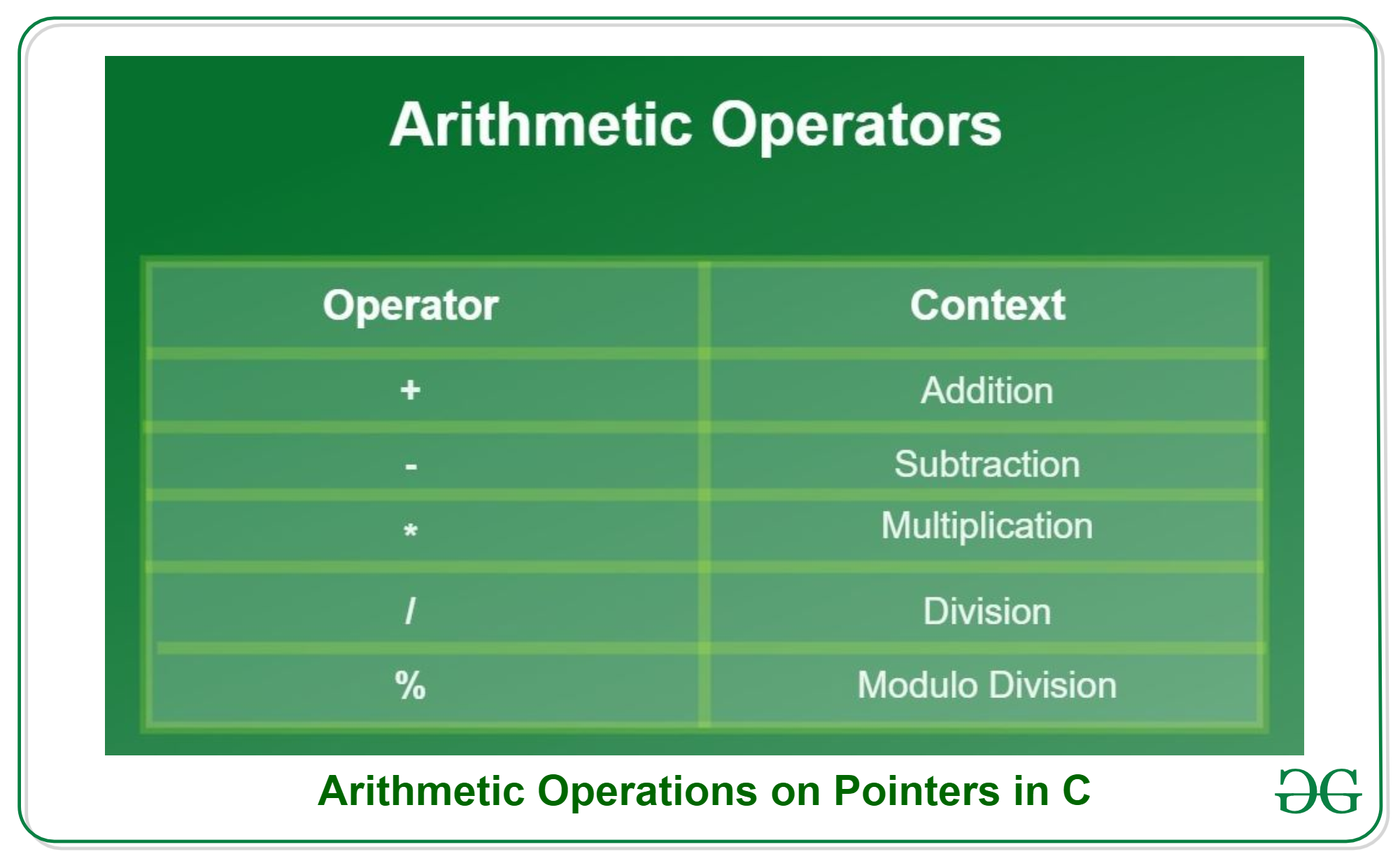Examples:

```*ptr1 + *ptr2
*ptr1 * *ptr2
*ptr1 + *ptr2 - *ptr3```

We can also directly perform arithmetic expressions on integers by dereferencing pointers. Let’s look at the example given below where p1 and p2 are pointers.

`*p1 + 10, *p2 - 5, *p1 - *p2 + 10, *p1/2 `

Below diagram represents how exactly the expression/operators work with pointers.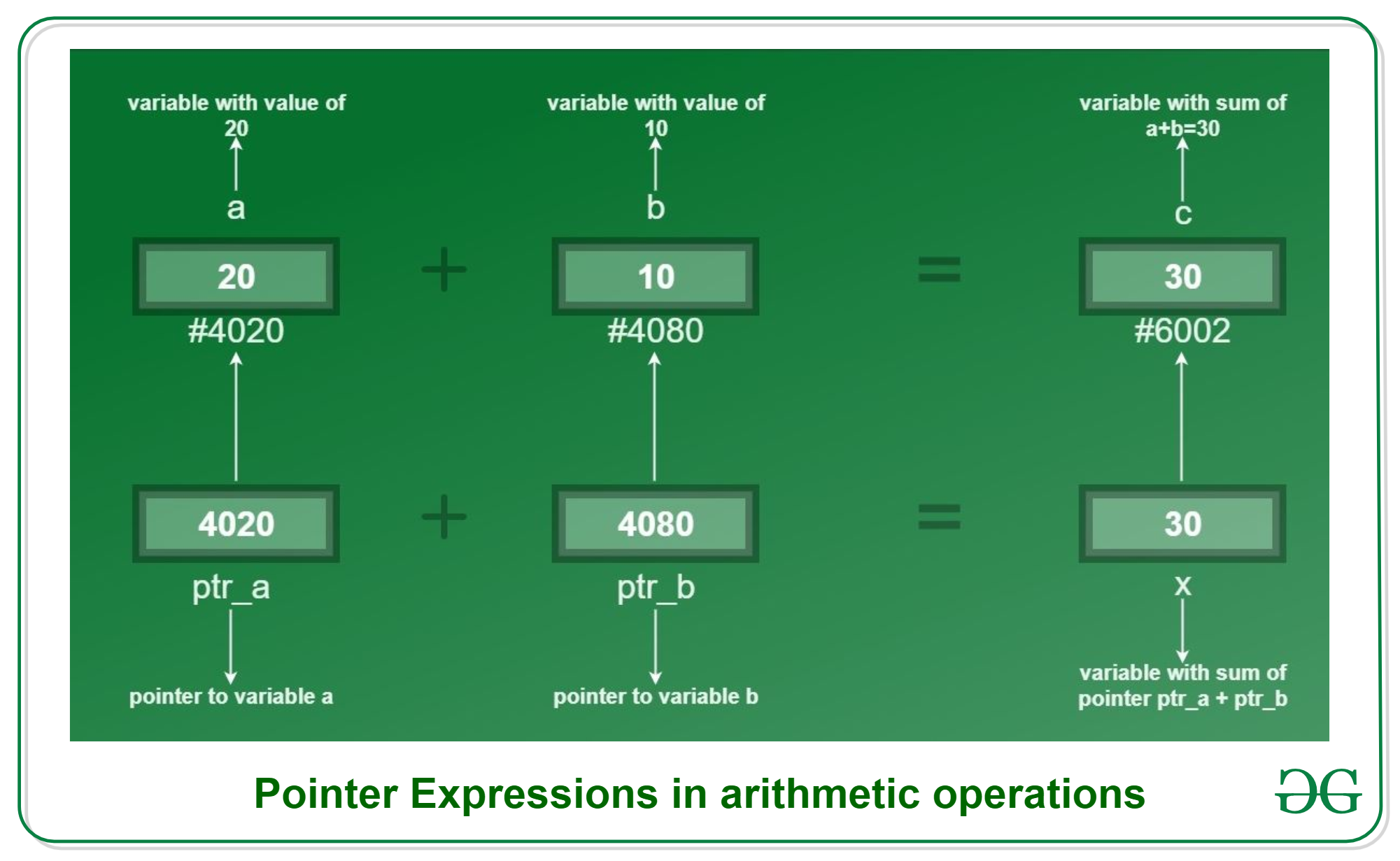As seen in the diagram, pointers ‘pa’ and ‘pb’ point to integer variables ‘a’ and ‘b’ respectively. The addition is performed directly between integer variables and pointer variable and the results are stored in integer variable ‘c’ and ‘x’ respectively. Both the results are the same. Let us understand pointer arithmetic expression better with given code:

## C

 `// Program showing pointer expressions` `// during Arithmetic Operations` `#include `   `int` `main()` `{` `    ``// Integer variables` `    ``int` `a = 20, b = 10;`   `    ``// Variables for storing arithmetic` `    ``// operations solution` `    ``int` `add, sub, ``div``, mul, mod;`   `    ``// Pointer variables for variables` `    ``// a and b` `    ``int` `*ptr_a, *ptr_b;`   `    ``// Initialization of pointers` `    ``ptr_a = &a;` `    ``ptr_b = &b;`   `    ``// Performing arithmetic Operations` `    ``// on pointers` `    ``add = *ptr_a + *ptr_b;` `    ``sub = *ptr_a - *ptr_b;` `    ``mul = *ptr_a * *ptr_b;` `    ``div` `= *ptr_a / *ptr_b;` `    ``mod = *ptr_a % *ptr_b;`   `    ``// Printing values` `    ``printf``("Addition = %d\n", add);` `    ``printf``("Subtraction = %d\n", sub);` `    ``printf``("Multiplication = %d\n", mul);` `    ``printf``("Division = %d\n", ``div``);` `    ``printf``("Modulo = %d\n", mod);` `    ``return` `0;` `}`

Output:

```Addition = 30
Subtraction = 10
Multiplication = 200
Division = 2
Modulo = 0```

Note: While performing division, make sure you put a blank space between ‘/’ and ‘*’ of the pointer as together it would make a multi-line comment(‘/*’). Example:

``` Incorrect:  *ptr_a/*ptr_b;
Correct:    *ptr_a / *ptr_b;
Correct:    (*ptr_a)/(*ptr_b);```

Relational Operators

Relational operations are often used to compare the values of the variable based on which we can take decisions. The given table shows the relational operators that can be performed on pointer variables.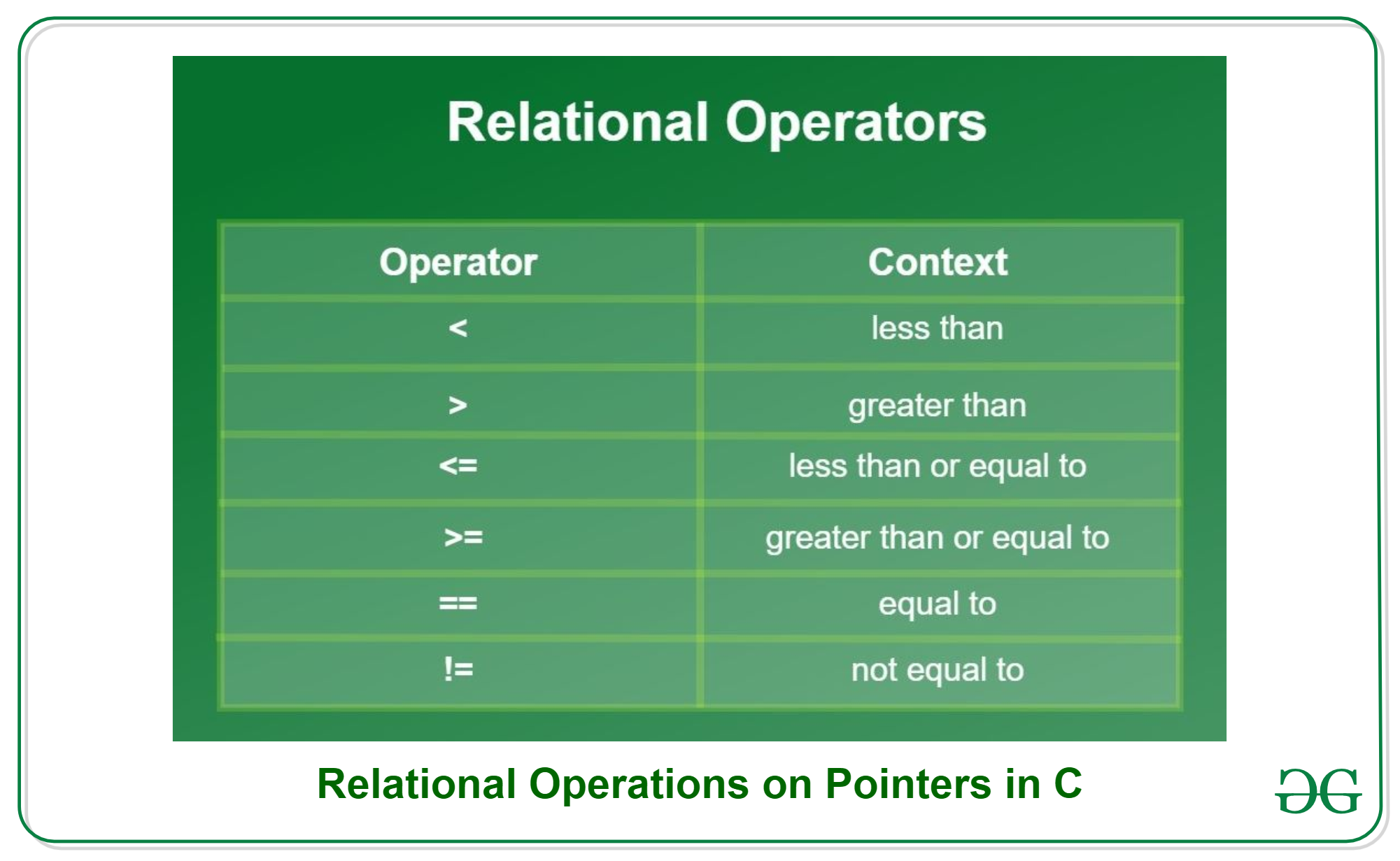Example:

```*ptr1 > *ptr2
*ptr1 < *ptr2```

The value of the relational expression is either 0 or 1 that is false or true. The expression will return value 1 if the expression is true and it’ll return value 0 if false. Let us understand relational expression on pointer better with the code given below:

## C

 `// Program showing pointer expressions` `// during Relational Operations` `#include ` `int` `main()` `{` `    ``// Initializing integer variables` `    ``int` `a = 20, b = 10;`   `    ``// Declaring pointer variables` `    ``int``* ptr_a;` `    ``int``* ptr_b;`   `    ``// Initializing pointer variables` `    ``ptr_a = &a;` `    ``ptr_b = &b;`   `    ``// Performing relational operations` `    ``// less than operator` `    ``if` `(*ptr_a < *ptr_b) {` `        ``printf``(` `            ``"%d is less than %d.", *ptr_a, *ptr_b);` `    ``}`   `    ``// Greater than operator` `    ``if` `(*ptr_a > *ptr_b) {` `        ``printf``(` `            ``"%d is greater than %d.", *ptr_a, *ptr_b);` `    ``}`   `    ``// Equal to` `    ``if` `(*ptr_a == *ptr_b) {` `        ``printf``(` `            ``"%d is equal to %d.", *ptr_a, *ptr_b);` `    ``}`   `    ``return` `0;` `}`

Output:

`20 is greater than 10.`

Output:

`20 is greater than 10.`

Assignment Operators

Assignment operators are used to assign values to the identifiers. There are multiple shorthand operations available. A table is given below showing the actual assignment statement with its shorthand statement.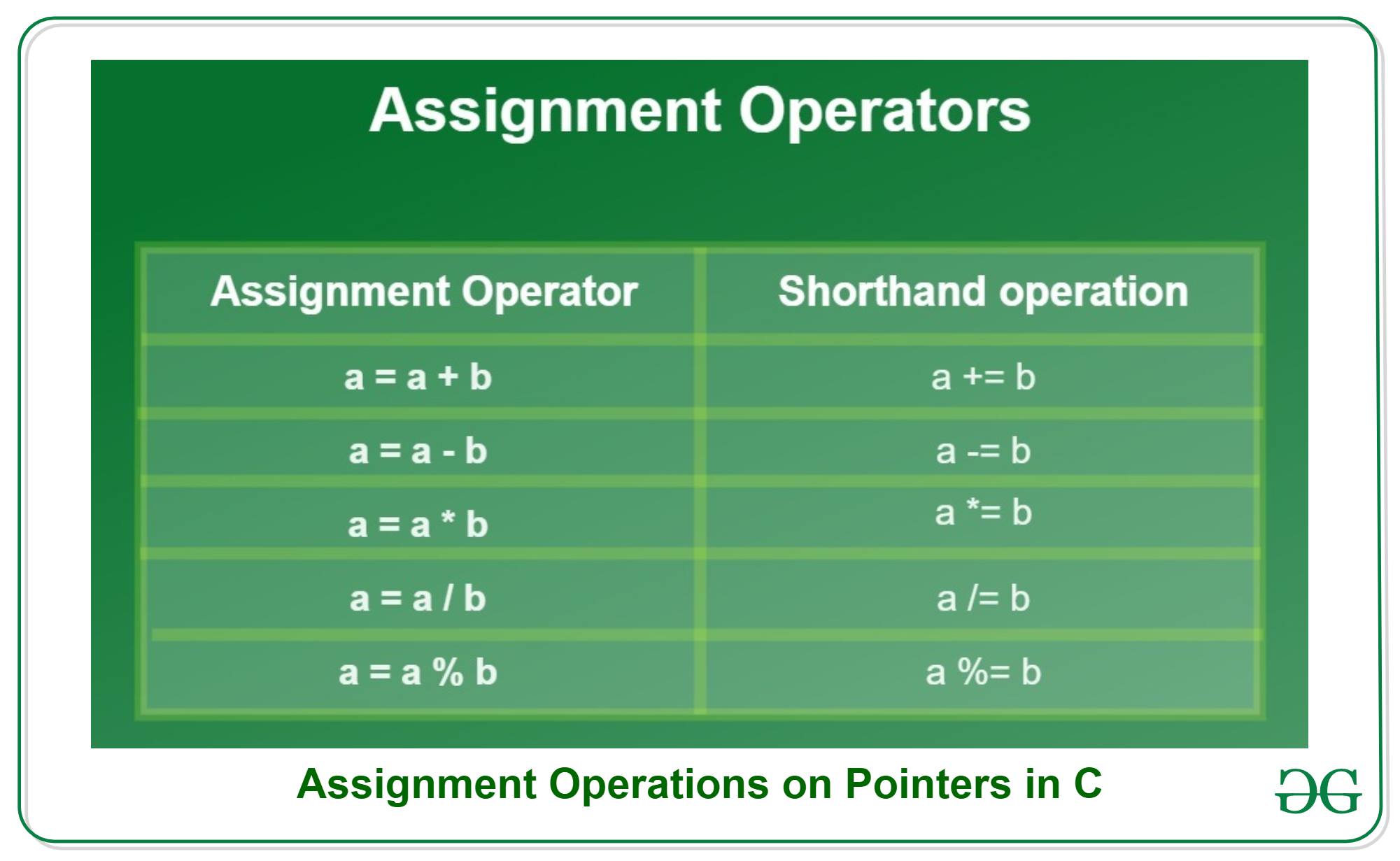Examples:

```*a=10
*b+=20
*z=3.5
*s=4.56743```

Let us understand assignment operator in better way with the help of code given below:

## C

 `// Program showing pointer expressions` `// during Assignment Operations` `#include ` `int` `main()` `{` `    ``// Initializing integer variable` `    ``int` `a = 30;`   `    ``// Declaring pointer variable` `    ``int``* ptr_a;`   `    ``// Initializing pointer using` `    ``// assignment operator` `    ``ptr_a = &a;`   `    ``// Changing the variable's value using` `    ``// assignment operator` `    ``*ptr_a = 50;`   `    ``// Printing value of 'a' after` `    ``// updating its value` `    ``printf``("Value of variable a = %d", *ptr_a);`   `    ``return` `0;` `}`

Output:

`Value of variable a = 50`

Conditional Operators

There is only one mostly used conditional operator in C known as Ternary operator. Ternary operator first checks the expression and depending on its return value returns true or false, which triggers/selects another expression. Syntax:

`expression1 ? expression2 : expression3;`

Example:

`c = (*ptr1 > *ptr2) ? *ptr1 : *ptr2;`
• As shown in example, assuming *ptr1=20 and *ptr2=10 then the condition here becomes true for the expression, so it’ll return value of true expression i.e. *ptr1, so variable ‘c’ will now contain value of 20.
• Considering same example, assume *ptr1=30 and *ptr2=50 then the condition is false for the expression, so it’ll return value of false expression i.e. *ptr2, so variable ‘c’ will now contain value 50.

Let us understand the concept through the given code:

## C

 `// Program showing pointer expressions` `// during Conditional Operations` `#include ` `int` `main()` `{` `    ``// Initializing integer variables` `    ``int` `a = 15, b = 20, result = 0;`   `    ``// Declaring pointer variables` `    ``int` `*ptr_a, *ptr_b;`   `    ``// Initializing pointer variables` `    ``ptr_a = &a;` `    ``ptr_b = &b;`   `    ``// Performing ternary operator` `    ``result = ((*ptr_a > *ptr_b) ? *ptr_a : *ptr_b);`   `    ``// Printing result of ternary operator` `    ``printf``("%d is the greatest.", result);` `    ``return` `0;` `}`

Output:

`20 is the greatest.`

Unary Operators

There are mainly two operators which are given as follows.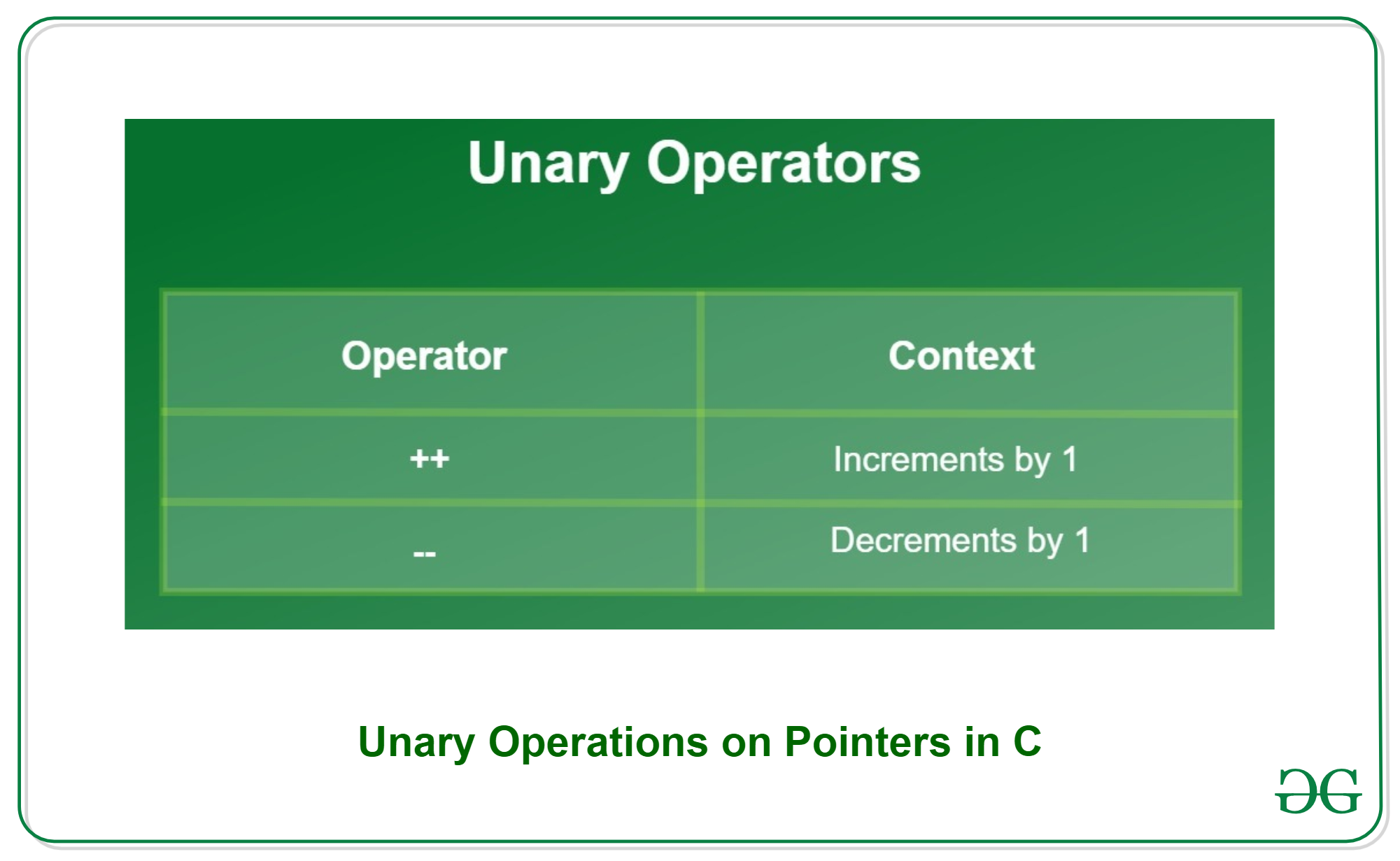Examples:

```(*ptr1)++
(*ptr1)--```

Let us understand the use of the unary operator through the given code:

## C

 `// Program showing pointer expressions` `// during Unary Operations` `#include ` `int` `main()` `{` `    ``// Initializing integer variable` `    ``int` `a = 34;`   `    ``// Declaring pointer variable` `    ``int``* ptr_a;`   `    ``// Initializing pointer variable` `    ``ptr_a = &a;`   `    ``// Value of a before increment` `    ``printf``("Increment:\n");` `    ``printf``(` `        ``"Before increment a = %d\n", *ptr_a);`   `    ``// Unary increment operation` `    ``(*ptr_a)++;`   `    ``// Value of a after increment` `    ``printf``(` `        ``"After increment a = %d", *ptr_a);`   `    ``// Value before decrement` `    ``printf``("\n\nDecrement:\n");` `    ``printf``(` `        ``"Before decrement a = %d\n", *ptr_a);`   `    ``// unary decrement operation` `    ``(*ptr_a)--;`   `    ``// Value after decrement` `    ``printf``("After decrement a=%d", *ptr_a);`   `    ``return` `0;` `}`

Output:

```Increment:
Before increment a = 34
After increment a = 35

Decrement:
Before decrement a = 35
After decrement a=34```

Bitwise Operators

Binary operators are also known as bitwise operators. It is used to manipulate data at bit level. Bitwise operators can’t be used for float and double datatype. A table is shown below with all bitwise operators: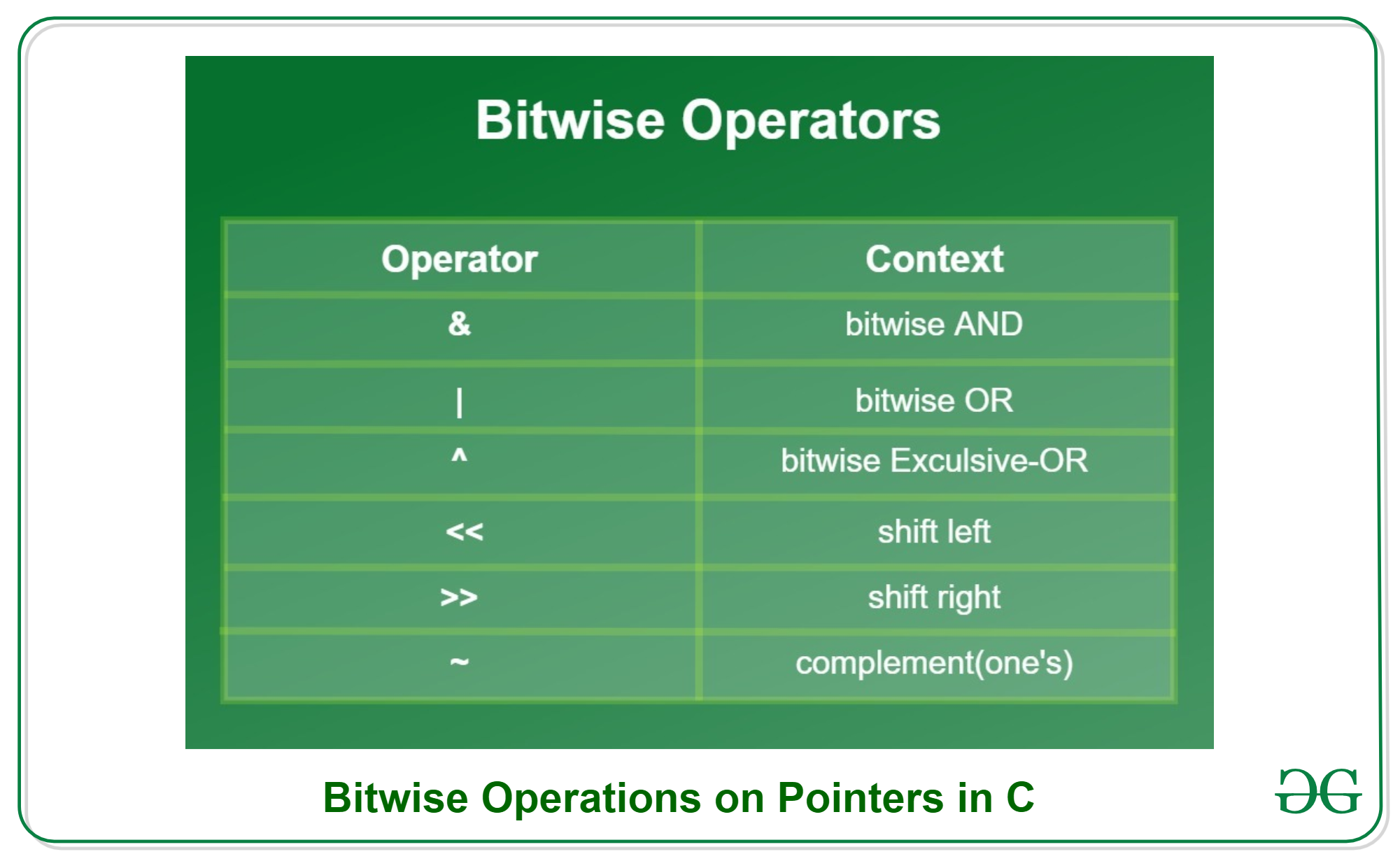Examples:

```*ptr1 & *ptr2
*ptr1 | *ptr2
*ptr1 ^ *ptr2```

Let us understand the concept through the given code:

## C

 `// Program showing pointer expressions` `// during Bitwise Operations` `#include ` `int` `main()` `{` `    ``// Declaring integer variable for` `    ``// storing result` `    ``int` `and, or, ex_or;`   `    ``// Initializing integer variable` `    ``int` `a = 1, b = 2;`   `    ``// Performing bitwise operations` `    ``// AND operation` `    ``and = a & b;`   `    ``// OR operation` `    ``or = a | b;`   `    ``// EX-OR operation` `    ``ex_or = a ^ b;`   `    ``// Printing result of operations` `    ``printf``("\na AND b = %d", and);` `    ``printf``("\na OR b = %d", or);` `    ``printf``("\na Exclusive-OR b = %d", ex_or);` `    ``return` `0;` `}`

Output:

```a AND b = 0
a OR b = 3
a Exclusive-OR b = 3```

My Personal Notes arrow_drop_up
Related Articles